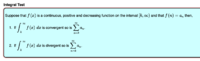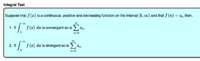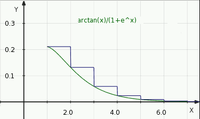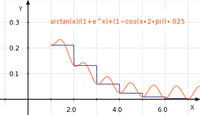# Convergence of an Integral

#### nasi112

##### Full Member
We all know that [MATH]\sum_{k=1}^{\infty} \frac{1}{k}[/MATH] diverges. One way to prove that by using the integral test.

This means, since the integral [MATH]\int_1^{\infty} \frac{1}{x} \ dx[/MATH] diverges, the sum is also diverges.

My question is what about the reverse? If the sum is converges, for example, does it mean the integral is also converges?

I am talking about a positive continuously decreasing function such as

[MATH]f(x) = \frac{\tan^{-1} x}{1 + e^x}, x \geq 1[/MATH]
Does this integral converge?

[MATH]\int_0^{\infty} \frac{\tan^{-1} x}{1 + e^x} \ dx[/MATH]
Can I say since [MATH]\sum_{k=0}^{\infty} \frac{\tan^{-1} k}{1 + e^k}[/MATH] converges, then the integral is also converges?

#### Cubist

##### Senior Member
Can I say since [MATH]\sum_{k=0}^{\infty} \frac{\tan^{-1} k}{1 + e^k}[/MATH] converges, then the integral is also converges?

I think you'd need to state, and prove, something like the following.

Since...
• [MATH]\sum_{k=\color{red}1\color{black}}^{\infty} f(k)[/MATH] converges
• AND f(x)>0 within the domain x≥1
• AND f(x) is continuous and decreasing within the domain x≥1
then [MATH]\int_{\color{red}1\color{black}}^{\infty} f(x) \ dx[/MATH] converges.

Think about f(x)=1−cos(2*pi*x). The sum is zero but the integral does not converge.

Last edited:
•topsquark, nasi112 and Jomo

#### Cubist

##### Senior Member
• [MATH]\sum_{k=\color{red}1\color{black}}^{\infty} f(k)[/MATH] converges
• AND f(x)>0 within the domain x≥1 <--------------------------------
• AND f(x) is continuous and decreasing within the domain x≥1

After thinking about it, the middle bullet is probably implied by the other two (and therefore unnecessary)

•nasi112 and Jomo

#### nasi112

##### Full Member
Why the reverse is also valid?

If the integral converges, then the sum is converges as well. This implies that the integral is larger than the sum. We will agree with this comparison.

But look here.

If the sum converges, then the integral converges as well. Why? The integral is larger. This comparison should be rejected. What do you say Cubist?

#### lex

##### Full MemberThat means that [MATH]\int_k^{\infty} f(x) dx[/MATH] exists [MATH]\Longleftrightarrow \sum_{n=k}^{\infty} a_n[/MATH] converges

•nasi112

#### nasi112

##### Full Member
View attachment 27274
That means that [MATH]\int_k^{\infty} f(x) dx[/MATH] exists [MATH]\Longleftrightarrow \sum_{n=k}^{\infty} a_n[/MATH] converges
lex
but the theorem did not mention if the sum converges, then the integral converges.

I am talking about the reverse. If you know that the sum converges, can you conclude that the integral is also convergent?

#### lex

##### Full Member
lex
but the theorem did not mention if the sum converges, then the integral converges.

I am talking about the reverse. If you know that the sum converges, can you conclude that the integral is also convergent?1. (Integral exists) [MATH]\Rightarrow[/MATH] (Series converges)
2. [MATH]\lnot[/MATH](Integral exists) [MATH]\Rightarrow \lnot[/MATH](Series converges)

2. Is logically equivalent to (Series converges) [MATH]\Rightarrow[/MATH] (Integral exists)

Do you know that
not A [MATH]\Rightarrow[/MATH] not B
is equivalent to
B [MATH]\Rightarrow[/MATH] A
?

So 1. and 2. together say: Integral exists if and only if series converges

•nasi112

#### nasi112

##### Full Member
Implication is a little confusing
but yeah thanks lex for the clarification. Now, it makes sense.

#### lex

##### Full Member
2. [MATH]\lnot[/MATH](Integral exists) [MATH]\Rightarrow \lnot[/MATH](Series converges)
The following statement is true:
IF the integral does not exist THEN the series does not converge

So if you find that the series converges, then it can't be true that the integral does not exist (since then the series would not converge).
So if you find that the series converges then the integral must exist.
IF the series converges THEN the integral exists

(all under the conditions of the integral test)

•nasi112

#### nasi112

##### Full Member
The following statement is true:
IF the integral does not exist THEN the series does not converge

So if you find that the series converges, then it can't be true that the integral does not exist (since then the series would not converge).
So if you find that the series converges then the integral must exist.
IF the series converges THEN the integral exists

(all under the conditions of the integral test)
wOW, as always, you are brilliant lex.

This is why when you read the truth table about implication, if A true and B false, the statement false. A is the controller here.

#### lex

##### Full Member
This is why when you read the truth table about implication, if A true and B false, the statement false. A is the controller here.
You could put it that way.

It's very useful to remember the logical equivalence of these two statements:

[MATH]A \Rightarrow B \\ \lnot B \Rightarrow \lnot A[/MATH]

•Cubist and nasi112

#### Subhotosh Khan

##### Super Moderator
Staff member
You could put it that way.

It's very useful to remember the logical equivalence of these two statements:

[MATH]A \Rightarrow B \\ \lnot B \Rightarrow \lnot A[/MATH]
That is called (if remember correctly) - contrapositive statement.

•Cubist, lex and nasi112

#### nasi112

##### Full Member
You could put it that way.

It's very useful to remember the logical equivalence of these two statements:

[MATH]A \Rightarrow B \\ \lnot B \Rightarrow \lnot A[/MATH]
Implication has always been my enemy, but I hope this time I overcome it.
Beautiful equivalence.

#### Cubist

##### Senior Member
I was thinking graphically when I wrote posts 2&3. See the following:-The area under the blue line is the sum (if x is extended to infinity). The area under the green line is the integral (again, if x extends). You can see that they will be different quantities. However, it's obvious that IF the series converges, then the integral must exist SINCE the green line is continuous and decreasing. That is, the green line is always below the blue "steps".

Below is an example where the function is not always decreasing. The series (area under the blue line) is equivalent to the graph above. However for this function the integral does not exist since the red line keeps jumping up between integer x values. Therefore, the area under the red line will tend towards infinity:-So it's important to state/ prove the "always decreasing" and "continuous" part of the argument. Or, to be more permissive, instead of "always decreasing" you need to show:- that for all x>c the function stays beneath the step function; and for all x≤c the function is finite. And c is just some finite constant.

•nasi112

#### Cubist

##### Senior Member
You could put it that way.

It's very useful to remember the logical equivalence of these two statements:

[MATH]A \Rightarrow B \\ \lnot B \Rightarrow \lnot A[/MATH]

Slightly off topic, but I must learn this notation. In computer studies and electronics we used one of these forms:-
• NOT D AND (E OR F)
• !D && (E || F)
• $$\bar{D}(E+F)$$

So, if A represents "series converges", B is "integral converges", C means "f(x) is continuous", and D means "f(x) decreasing" !

[MATH]A \wedge C \wedge D \Rightarrow B[/MATH]
[MATH] \lnot( B ) \Rightarrow \lnot(A \wedge C \wedge D) [/MATH]
[MATH] \lnot B \Rightarrow \lnot A \lor \lnot C \lor \lnot D [/MATH]
(the integral doesn't converge) IMPLIES (the series doesn't converge) OR (the function isn't continuous) OR (function not decreasing)

I might be too old to learn this notation. It currently looks like modem noise to me #^£54634$$!•nasi112 #### lex ##### Full Member I might be too old to learn this notation. It currently looks like modem noise to me #^£54634$$ !See! We might feel too old, but it's never too late!
(Not wishing to add to the alphabet soup, we also want f non-negative)!

•Cubist and nasi112

#### nasi112

##### Full Member
I was thinking graphically when I wrote posts 2&3. See the following:-

View attachment 27276

The area under the blue line is the sum (if x is extended to infinity). The area under the green line is the integral (again, if x extends). You can see that they will be different quantities. However, it's obvious that IF the series converges, then the integral must exist SINCE the green line is continuous and decreasing. That is, the green line is always below the blue "steps".

Below is an example where the function is not always decreasing. The series (area under the blue line) is equivalent to the graph above. However for this function the integral does not exist since the red line keeps jumping up between integer x values. Therefore, the area under the red line will tend towards infinity:-
View attachment 27277

So it's important to state/ prove the "always decreasing" and "continuous" part of the argument. Or, to be more permissive, instead of "always decreasing" you need to show:- that for all x>c the function stays beneath the step function; and for all x≤c the function is finite. And c is just some finite constant.
Thanks a lot Cubist for illustrating those two graphical examples.
I was confused at first because there are a lot of rules to consider
but thanks for lex for giving me some examples and thanks to you Cubist for taking the time to write and graph functions
it makes sense now why the reverse is also true

Slightly off topic, but I must learn this notation. In computer studies and electronics we used one of these forms:-
• NOT D AND (E OR F)
• !D && (E || F)
• $$\bar{D}(E+F)$$

So, if A represents "series converges", B is "integral converges", C means "f(x) is continuous", and D means "f(x) decreasing" !

[MATH]A \wedge C \wedge D \Rightarrow B[/MATH]
[MATH] \lnot( B ) \Rightarrow \lnot(A \wedge C \wedge D) [/MATH]
[MATH] \lnot B \Rightarrow \lnot A \lor \lnot C \lor \lnot D [/MATH]
(the integral doesn't converge) IMPLIES (the series doesn't converge) OR (the function isn't continuous) OR (function not decreasing)

I might be too old to learn this notation. It currently looks like modem noise to me #^£54634 !with your examples, things become clear, but I don't know why when I read implication on other examples, I get confused.

•Cubist